nextnano.com  nextnano³  Download | Search | Copyright | Publications  * password protected nextnano³ softwareBIO-1D Protein sensor

# nextnano3 - Tutorial

## ISFET (Ion-Selective Field Effect Transistor): Detection of charged proteins with a SOI (silicon-on-insulator) electrolyte sensor

Author: Stefan Birner

If you want to obtain the input file that is used within this tutorial, please submit a support ticket.
```-> 1DSOI_electrolyte_sensor.in ```Acknowledgement: The author - Stefan Birner - would like to thank Christian Uhl and Michael Bayer for helping to include the electrolyte features into nextnano³.

## ISFET (Ion-Selective Field Effect Transistor): Detection of charged proteins with a SOI (silicon-on-insulator) electrolyte sensor

This tutorial is based on the following paper:

Theoretical model for the detection of charged proteins with a silicon-on-insulator sensor
S. Birner, C. Uhl, M. Bayer, P. Vogl
J. Phys.: Conf. Ser. 107, 012002 (2008)

The general idea of this paper has been presented already in the diploma thesis of Christian Uhl, Charge Transport at Functionalized Semiconductor Surfaces, TU Munich (2004).

The simulations are based on previously performed experiments:

Field Effect of Screened Charges: Electrical Detection of Peptides and Proteins by a Thin-Film Resistor
S.Q. Lud, M.G. Nikolaides, I. Haase, M. Fischer, A.R. Bausch
ChemPhysChem 7, 379 (2006)

Summary

For a bio-sensor device based on a silicon-on-insulator (SOI) structure, we calculate the sensitivity to specific charge distributions in the electrolyte solution that arise from protein binding to the semiconductor surface. This surface is bio-functionalized with a lipid layer so that proteins can specifically bind to the headgroups of the lipids on the surface. We consider charged proteins such as the green fluorescent protein (GFP) and artificial proteins that consist of a variable number of aspartic acids. Specifically, we calculate self-consistently the spatial charge and electrostatic potential distributions for different ion concentrations in the electrolyte. We fully take into account the quantum mechanical charge density in the semiconductor. We determine the potential change at the binding sites as a function of the protein charge and ionic strength. Comparison with experiment is generally very good. Furthermore, we demonstrate the superiority of the full Poisson-Boltzmann equation by comparing its results to the simplified Debye-Hückel approximation.

We discuss a silicon-on-insulator (SOI) based thin-film resistor that we will model in detail in this tutorial. Indeed, such a device has been realized experimentally for chemical and biological sensor applications. Peptides with a single charge can be detected and it is possible to distinguish single charge variations of the analytes even in physiological electrolyte solutions.

Figure 1 shows the layout of this bio-functionalized silicon-on-insulator device. It consists of a SiO2-Si-SiO2 structure. Specifically, we take a silicon dioxide buffer layer with a thickness of 200 nm (0 nm - 200 nm) and a conducting silicon layer of 30 nm (200 nm - 230 nm) which is homogeneously p-type doped with boron (doping density p = 1 * 1016 cm-3). The silicon layer is covered by a native SiO2 layer with a thickness of 2 nm (230 nm - 232 nm).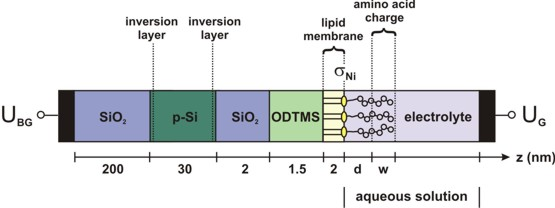Fig. 1: Schematic layout of the considered SOI structure.
There is a negative interface charge density sigmaNi at the lipid/electrolyte interface.
The amino acid charge is assumed to be distributed homogeneously over a width w.
The electrolyte region includes the histidine-tagged amino acids as well as the neutral part of the tag of length d.

This oxide layer is passivated by an ODTMS (octadecyltrimethoxysilane) monolayer which is required for the bio-functionalization of the semiconductor device. We take a 1.5 nm thick oxide-like ODTMS layer (232 nm - 233.5 nm) and use a static dielectric constant of epsilon = 1.5. Due to the passivation by ODTMS, we assume that no interface charges are present at the native oxide surface. The ODTMS layer is surface-functionalized with a lipid membrane that allows the specific binding of molecules. This lipid monolayer (2 nm) consists of DOGS-NTA (1,2-dioleoyl-sn-glycero-3-{[N(5-amino-1-carboxypentyl)iminodiacetic acid]succinyl}) incorporated into two matrix lipids (DMPC (1,2-dimyristoyl-sn-glycero-3-phosphocholine) and cholesterol). The lipid membrane is treated as an insulator using the same material parameters as for ODTMS. Thus, no charge carriers are assumed to be present within this layer. As the lipid layer is very dense, no electrolyte is considered within the lipid region.

The functionalized surface exposes NTA headgroups that carry two negative charges to the electrolyte solution. They have the ability to form a chelate complex with nickel ions if the latter are present in the solution. Upon loading with nickel, the charge of the headgroup changes by +1e and is then considered to be -1e. This results in a negative sheet density sigmaNi at the lipid/electrolyte interface. The surface density of the DOGS-NTA lipids is considered to be 5 % (fNTA = 0.05). The approximated headgroup area, i.e. the average area per functional DOGS-NTA is assumed to be ANTA = 0.65 nm2. Consequently, the density of the headgroups is sNTA = fNTA / ANTA = 7.7 * 1012 cm-2, so that the resulting charge density sigmaNi is given by sigmaNi = - e * sNTA, where we have assumed that each headgroup carries one negative charge upon exposure to Ni.

• Interface 5 (235. nm): Fixed interface charge sigmaNi. More details: ``` \$interface-states```
``` \$interface-states   state-number          = 1            ! between lipid membrane / electrolyte at 235.5 nm   state-type            = fixed-charge ! sigma_Ni = - e * s_NTA; s_NTA = 7.7 * 10^12 cm^-2   interface-density     = -7.7d12      ! - 7.7 * 10^12 [|e|/cm^2]```

Output: The file``` densities/interface_densitiesD.txt ```gives us information about the relevant interface charge densities.

For the ionic content of the electrolyte we consider a variable concentration of KCl (i.e. 10, 50, 90 or 140 mM), and a fixed concentration of 1 mM NiCl2 and 1 mM of phosphate buffer saline (PBS) solution, respectively. The NiCl2 dissociates into 1 mM of doubly charged cations and 2 mM of singly charged anions.

For all calculations, the pH of the bulk electrolyte has been set to 7.5.

``` \$electrolyte   ...   pH-value              = 7.5d0       ! pH = -lg(concentration) = 7.5 -> concentration in [M]=[mol/l]```

The calculated concentrations of the PBS buffer ions are listed in Table 1 for different salt concentrations. These values refer to the bulk electrolyte. In the vicinity of the semiconductor surface and in regions around charged analytes, however, the actual concentrations of the buffer ions vary locally. Our buffer model automatically takes this into account because the spatial variations of pH, ionic strength and pKa,T' are determined self-consistently (see Appendix B of the above mentioned paper for more details). The ionic strengths of the electrolyte solutions considered in this work, are largely dominated by the respective concentrations of singly charged anions and cations from KCl as can be seen in Table 1. In these particular cases, i.e. small concentrations of PBS with respect to KCl, the Debye screening length is fully dominated by the KCl concentration.The electrolyte region (235.5 nm - 999 nm) contains the following ions (plus the buffer ions!):
```  !-----------------------------------------------------------------------------!  ! The electrolyte (NiCl2, KCl) contains four types of ions:  !   1)    2 mM singly charged anions  (1-)    <-   1 mM NiCl2  !   2)    1 mM doubly charged cations (2+)    <-   1 mM NiCl2  !   3a)  50 mM singly charged anions  (Cl-)   <-  50 mM KCl  !   3b) 140 mM singly charged anions  (Cl-)   <- 140 mM KCl  !   4a)  50 mM singly charged cations (K+)    <-  50 mM KCl  !   4b) 140 mM singly charged cations (K+)    <- 140 mM KCl  ! In addition, the buffer itself provides further ions.  !-----------------------------------------------------------------------------!  \$electrolyte-ion-content  !------------------------------  !   1 mM NiCl2  !------------------------------   ion-number        = 1              ! ```2 mM singly charged anions```   ion-name          = Cl-            !   ion-valency       = -1d0           ! ```charge of the ion:``` 1-   ion-concentration =   2d-3         ! ```Input in units of:``` [M] = [mol/l] = 1d-3 [mol/cm³]   ion-region        = 235.5d0  999d0 ! ```refers to region where the electrolyte has to be applied to```   ion-number        = 2              ! ```1 mM doubly charged cations```   ion-name          = Ni2+           !   ion-valency       =  2d0           ! ```charge of the ion:``` 2+   ion-concentration =   1d-3         ! ```Input in units of:``` [M] = [mol/l] = 1d-3 [mol/cm³]   ion-region        = 235.5d0  999d0 ! ```refers to region where the electrolyte has to be applied to
```  !------------------------------  ! a)  50 mM KCl  ! b) 140 mM KCl  !------------------------------   ion-number        = 3              ! ```140 or 50 mM singly charged anions```   ion-name          = Cl-            !   ion-valency       = -1d0           ! ```charge of the ion:``` Cl- ! ion-concentration =  50d-3         ! ```Input in units of:``` [M] = [mol/l] = 1d-3 [mol/cm³]   ion-concentration = 140d-3         ! ```Input in units of:``` [M] = [mol/l] = 1d-3 [mol/cm³]   ion-region        = 235.5d0  999d0 ! ```refers to region where the electrolyte has to be applied to

```  ion-number        = 4              ! ```140 or 50 mM singly charged cations```   ion-name          = K+             !   ion-valency       =  1d0           ! ```charge of the ion:``` K+ ! ion-concentration =  50d-3         ! ```Input in units of:``` [M] = [mol/l] = 1d-3 [mol/cm³]   ion-concentration = 140d-3         ! ```Input in units of:``` [M] = [mol/l] = 1d-3 [mol/cm³]   ion-region        = 235.5d0  999d0 ! ```refers to region where the electrolyte has to be applied to

In addition to these four types of ions, the pH value (as specified in` \$interface-states`) automatically determines inside the code four further types of ions, namely the concentration of H3O+, OH`-` and the corresponding anions`-`  (conjugate base; zero in this tutorial because pH = 7.5 > 7) and cations+ (conjugate acid: [cation`+`] = 10-pOH - 10-pH = 10-6.5 - 10-7.5 = 2.85 x 10-7). For details, confer` \$electrolyte-ion-content`.

``` !---------------------------------------------------------------------------!  ! Note that the site-binding-model is not used in our calculation.  ! Thus the interface-density is set to zero.  !---------------------------------------------------------------------------!  \$interface-states   ...   state-number          = 2           ! between lipid membrane / electrolyte at 235.5 nm   state-type            = electrolyte ! sigma_adsorbed   interface-density     = 0d0         ! no site-binding model!! ! [cm-2] - ``` total density of surface sites, i.e. surface hydroxyl groups```   adsorption-constant   = 1d0         ! no site-binding model!! ! K_1 = adsorption constant   dissociation-constant = 1d0         ! no site-binding model!! ! K_2 = dissociation constant  ```

In addition to the previously described ions, additional ions occur due to the buffer. Here, a PBS buffer (phosphate buffer) is used with a concentration of 1 mM.

We calculate the concentration of the buffer ions automatically depending on temperature, ionic strength, pH value, pKa,T' value(s) and spatial coordinate.

For that reason, four additional types of ions are present: H2PO4`-`, HPO42`-`, PO43`-`, Na+
The concentration of these buffer ions is calculated automatically according to the specifiers that are set below. For details, confer the descriptions of the keywords` \$electrolyte ` and``` \$buffer-solutions```.

``` \$electrolyte   ...   include-buffer                = yes    !   buffer-name                   = PBS    ! ```PBS (phosphate buffer)```   buffer-concentration          =  1d-3  !  ```1 mM PBS [M] = [mol/l] = [mole/liter]```   pKa-temperature-dependence    = yes   pKa-ionic-strength-dependence = yes   pKa-spatial-dependence        = yes  ```

## Electrode potential UG

In order to allow for comparison with experiment, we need to determine the potential of the reference electrode in the electrolyte. We consider a SOI structure that is operated in inversion. This means that the p-doped Si channel is inverted and becomes n-type. The electrode in the solution is assumed to determine the bulk electrolyte potential UG. In this example the applied gate voltage is UG = 2.2 V. This value yields channel densities of the order of a few 1011 cm-2 depending on the actual configuration of the system in terms of ion concentrations and protein charges. The experiment also yields electron densities of this magnitude. We note that the electrode potential UG does not reflect the experimentally applied potential since we do not take into account potential drops at the electrode. UG is defined on a scale where the Fermi energy in the semiconductor determines zero potential.

We have to solve the nonlinear Poisson equation over the whole device, i.e. including the Poisson-Boltzmann equation that governs the charge density in the electrolyte region.
As for the boundary conditions we assume at the right boundary (Electrolyte/Metal) a Dirichlet boundary condition where the electrostatic potential phi is equal to UG where UG is the gate voltage determined by an electrode in the electrolyte solution and which is constant throughout the entire electrolyte region. Note that the reference potential UG enters the Poisson-Boltzmann equation and also the equation for the site-binding model at the oxide/electrolyte interface. The latter is not used here, however. So the Dirichlet boundary condition is phi = 2.2 V. This corresponds to the fact that at the right part of the electrolyte,  i.e. at 'infinity' (at 9999 nm) the ion concentration is the 'equilibrium' (default) concentration as defined in``` \$electrolyte-ion-content```.
At the left boundary (Metal/SiO2) we use a Neumann boundary condition with a zero potential gradient that corresponds to an electric field E = 0 V/m.

```  \$poisson-boundary-conditions    poisson-cluster-number  = 1    region-cluster-number   = 1    boundary-condition-type = Neumann    electric-field          = 0d0      ! 0 [V/m]    poisson-cluster-number  = 2    region-cluster-number   = 7    boundary-condition-type = Dirichlet    potential               = 2.2d0    ! phi = 2.2 [V] <=> UG = 2.2 [V]```

We simulate the structure along the z direction so that the structure is effectively one-dimensional, i.e. laterally homogeneous.
The heterostructure is assumed to be grown along the  direction and is not strained.

## Protein charge distribution

We study a protein charge distribution that is spatially separated from the lipid membrane due to a neutral tag in between the protein and the lipids. The protein is modeled to allow for comparison with experiment. We consider an artificial protein structure where amino acids are tagged to a histidine chain. A part of this artificial protein remains uncharged since no amino acids get attached there. The width of this part has been taken to be 1.5 nm. By contrast, the rest of the histidine backbone is negatively charged since we consider aspartic acids (ASP) that carry one negative charge each for the binding to the tag. The electrolyte region starts at the membrane surface and includes the regions of both the neutral part of the tag and the protein charge distribution so that the ions in the aqueous solution screen the protein charge. Fig. 1 illustrates the structure as considered for the calculations. The artificial protein binds to an NTA headgroup on the membrane. It is possible to manufacture the protein consisting of his-tagged aspartic acids (ASP) that bind to the tag. The integrated charge density in the protein region therefore changes in magnitudes of` -` e sigmaNTA by increasing the number of ASP. The width of the charged part can be assumed to be variable but we used 1.5 nm in this example.

We represent the aspartic acids by a doping function.

``` \$doping-function   ...   !---------------------------------------------------------------------------   ! 1 / 65 Angstrom^2 * 5 % = 7.6923 * 10-16 m2 = 7.6923 * 1012 cm-2   !---------------------------------------------------------------------------   !-----------------------------------------------------------------------------------   ! volume charge = - e * sigma_NTA      / 1.5 nm         =   !               = - e * 7.7 * 1012 cm-2 / 1.5 * 10^-7 cm = - e * 51.3333 * 1018 cm-3   !----------------------------------------------------------------------------------   doping-function-number      = 2               ! simga_NTA = 7.7 * 1012 cm-2   impurity-number             = 2 ! doping-concentration        = 0d0             ! ```number of aspartic asics``` =  0 =>    0             ! 1 * 1018 cm-3   doping-concentration        =  51.333333333d0 ! ```number of aspartic asics``` =  1 => -  1 e sigma_NTA ! 1 * 1018 cm-3 ! doping-concentration        = 102.666666666d0 ! ```number of aspartic asics``` =  2 => -  2 e sigma_NTA ! 1 * 1018 cm-3 ! doping-concentration        = 205.333333333d0 ! ```number of aspartic asics``` =  4 => -  4 e sigma_NTA ! 1 * 1018 cm-3 ! doping-concentration        = 308d0           ! ```number of aspartic asics``` =  6 => -  6 e sigma_NTA ! 1 * 1018 cm-3 ! doping-concentration        = 410.666666666d0 ! ```number of aspartic asics``` =  8 => -  8 e sigma_NTA ! 1 * 1018 cm-3 ! doping-concentration        = 513.333333333d0 ! ```number of aspartic asics``` = 10 => - 10 e sigma_NTA ! 1 * 1018 cm-3   only-region                 = 237.5d0 239d0   ! ```negative protein charge distribution (width 1.5 nm)``` ```Alternatively one could do the following doping sweep:
``` doping-concentration         = 0d0             ! ```number of aspartic asics``` =  0 =>    0             ! 1 * 1018 cm-3  doping-sweep-active          = yes             ! ```for doping sweep```  doping-sweep-step-size       = 51.333333333d0  ! ```for doping sweep, doping concentration in units of` 1 * 1018 cm-3`
```  doping-sweep-number-of-steps = 10              ! ```for doping sweep```  \$impurity-parameters   ...   impurity-number             = 2 ! impurity-name               = aspartic-acid   impurity-type               = p-type   number-of-energy-levels     = 1   energy-levels-relative      = -1000d0        ! [eV] ```"all ionized"```   degeneracy-of-energy-levels = 1```

## Conduction band edge and electron density

Since we have specified all about the sensor and the proteins in the electrolyte, we are now ready to calculate the electrostatic potential in the semiconductor/electrolyte system for several protein charge distributions. The quantum mechanical charge densities are calculated self-consistently by solving the Schrödinger equation in the silicon channel. The Schrödinger and Poisson equations are coupled via the electrostatic potential and the charge densities.

Figure 2 shows the calculated conduction band edge and the electron density in the silicon channel for a backgate voltage of UBG = 25 V. Indicated is also the position of the Fermi level EF and the electrostatic potential. Specifying a value for the potential UG of the reference electrode is equivalent to a Dirichlet boundary condition for the electrostatic potential of the Poisson-Boltzmann equation. An increase of UG leads to higher electron densities in the right channel. Therefore, the variation of UG and the backgate voltage UBG allows one to increase the sensitivity of the sensor by adjusting the ratio of the densities of the two channels. Our calculations yield channel densities of the order of a few 1012 cm`-`2. They are modulated slightly by the actual configuration of the system in terms of ion concentrations and protein charges. Since a lower surface potential phis yields a lower electron density in the inverted silicon channel, the source-drain current is expected to decrease if negatively charged proteins bind to the functionalized sensor surface.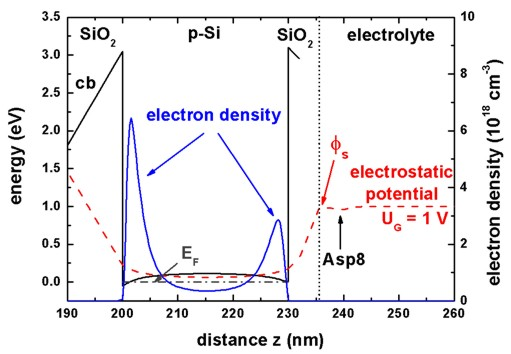Fig. 2: Calculated conduction band edge (black solid line) and electrostatic potential (dashed line) for the SOI structure at 50 mM KCl.
The electron charge density (blue solid line) of the two inversion layers is shown.
In the semiconductor, the Fermi level is set constant at EF = 0 eV.
Upon binding to the lipid membrane, the charge of the aspartic acid (Asp8) modifies the surface potential phis.
The interface between the lipid membrane and the electrolyte is indicated by the vertical dotted line.

## Electrostatic potential for different numbers of aspartic acids

We calculated the potential and charge distributions in the semiconductor/electrolyte structure self-consistently for different numbers of aspartic acids. Importantly, variations of the protein charge distribution that affect the potential in the SOI structure are calculated. Since the potential at the lipid/electrolyte interface sets the boundary value for the spatial potential distribution in the semiconductor, we determine the lipid/electrolyte interface potential and its changes as a function of the respective parameter that is varied. The interface potential is also referred to as the surface potential since the lipid layer constitutes the surface of the semiconductor.

The magnitude of the negative protein charge density increases with the number of aspartic acids. This results in a lower potential in the protein region. Fig. 3 shows the calculated potential distribution for a varying number of aspartic acids where the surface potential decreases with increasing protein charge. The pH value of the electrolyte has been set to 7.5 and the concentration of KCl in the solution has been assumed to be 140 mM. As already mentioned, the neutral part of the histidine tag is assumed to be 1.5 nm. The respective protein charges that are considered have been homogeneously distributed over 1.5 nm and are indicated by the shaded region in Fig. 3. We have considered the chemical structure of the histidine tagged amino acids in order to obtain this parameter. We note, that the electrolyte region starts at the lipid surface at 236 nm.

Now we want to plot the electrostatic potential for different numbers of aspartic acids.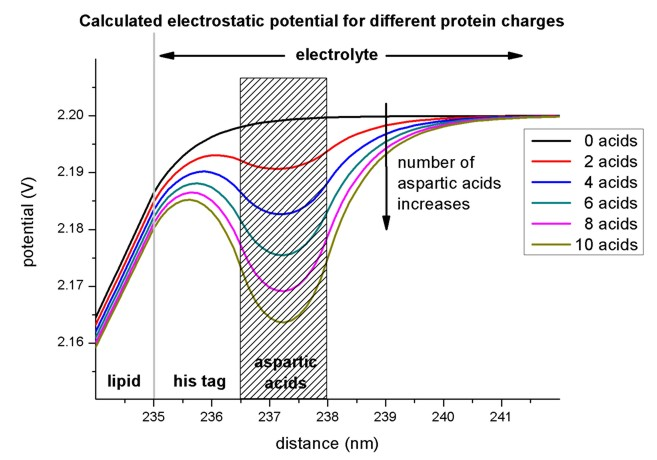Fig. 3: Spatial electrostatic potential distribution for pH = 7.5 in the electrolyte by using the nonlinear Poisson-Boltzmann (PB) ion distribution for different numbers of aspartic acids.
(Electrolyte: 10 mM phosphate buffer, 1 mM NiCl2, 140 mM KCl, UG = 2.2 V)

## Surface potential change

We calculated the potential change at the lipid membrane as a function of the number of aspartic acids that are attached to each histidine tag for KCl concentrations of 50 mM and 140 mM. The reference level phiref,surface for the surface potential change scale is the one for zero protein charge. We define the surface potential change Delta_phi by

Delta_phi(NASP) = phiref,surface - phi(NASP)

where NASP denotes the number of aspartic acids. A positive potential change therefore implies that the reference level is higher compared to the situation with a nonzero number of aspartic acids. The results are shown in Fig. 4. Due to the lower ion concentrations in the case of 50 mM KCl, the protein charge density is less efficiently screened. Consequently, the surface potential change is larger compared to the case of 140 mM KCl. Therefore the variations in the charge density in the silicon channel are greater in the case of 50 mM KCl.

The Debye screening lengths of the two different buffer solutions were calculated as follows:
`-  `50 mM KCl: 1.093 nm (for 10 mM PBS buffer)
`- `140 mM KCl: 0.746 nm (for 10 mM PBS buffer)
`-  `50 mM KCl: 1.303 nm (for   1 mM PBS buffer)
`- `140 mM KCl: 0.805 nm (for   1 mM PBS buffer)

The right figure shows the different sensitivity due to the PBS buffer concentrations. Using a 1 mM PBS buffer reduces the ionic strength and thus the screening of the aspartic acid charges with respect to the 10 mM PBS buffer.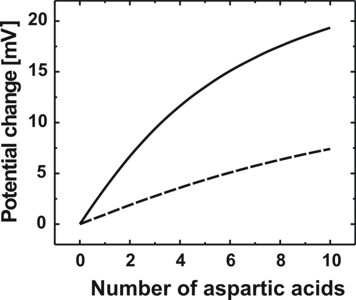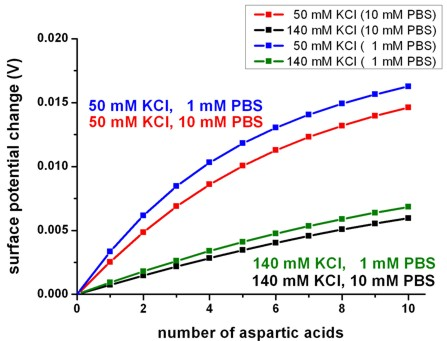Fig. 4: Calculated surface potential change at the lipid membrane as a function of the number of aspartic acids in the artificial protein. The solid line (or black line) depicts the case of a KCl concentration of 50 mM whereas the dashed line (or red line) represents the case of 140 mM of KCl in the electrolyte solution.
(Left figure: Electrolyte: 10 mM phosphate buffer, 1 mM NiCl2, UG = 2.2 V)

## Influence of the ionic strength on the sensitivity

### Green fluorescent protein

As a second protein, we consider the binding of the so-called green fluorescent protein (GFP) to the lipid membrane. GFP is also histidine-tagged to the NTA headgroups of the membrane. The size of GFP is larger (length of 4-5 nm) compared to his-tagged aspartic acids. We assume a charge distribution of width w = 3.0 nm that is connected with a neutral tag of width d = 2.3 nm to the NTA headgroups. At pH = 7.5, GFP carries eight negative charges that we assume to be homogeneously distributed over the protein region w.

Here, we use the same sensor structure as in Fig. 1 but detect another protein. We consider the specific binding of the green fluorescent protein (GFP) to the lipid membrane and calculate the change of the surface potential as a function of the salt concentration (10, 50, 90 or 140 mM KCl) in the electrolyte. At pH = 7.5, GFP carries a net negative charge of -8e as can be derived from the primary structure if one calculates the charge of the sidechains for the used buffer solution. Consequently, the integrated charge density of GFP is given by sigmaGFP = - 8 e sNTA. We assume for simplicity that this charge is distributed evenly over a distance of wGFP = 3 nm which is close to the length of the GFP (4-5 nm). Based on the previous section, the length of the neutral part of the tag has been taken to be d = 2.3 nm. Here, this length has been assumed to be the same for all KCl concentrations.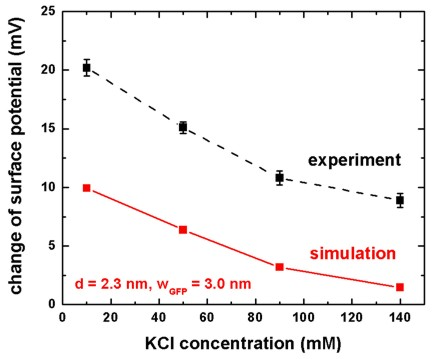Fig. 5: Calculated change of the surface potential as a function of the KCl concentration
where we have assumed a charge distribution that resembles the green fluorescent protein.
Included are experimental data of the above cited reference.
The lines are a guide to the eye.

We have calculated the change of the surface potential as a function of the KCl concentration in the electrolyte. The electrolyte has the same properties as for the aspartic acids (1 mM PBS, 1 mM NiCl2). Figure 5 shows the results and compares them to the experimental data of the above cited reference. The trend of the influence of the ionic strength on the sensitivity is well reproduced by our calculations. We note that the exact orientation of the GFP molecule at the surface is not known. A slight tilt angle can increase the measured sensor response due to the exponential dependence of the signal from the distance of the charges. The reduction of the spacing d or the
width wGFP of the charge distribution leads to larger surface potential changes. At higher ion concentrations, the Debye screening length of the electrolyte decreases, and thus the charges of the protein are more effciently screened by the ions in the electrolyte. This leads to a reduced sensitivity which is approximately linear to the inverse of the Debye screening length. The Debye screening lengths of the different salt concentrations are listed in Table 1.